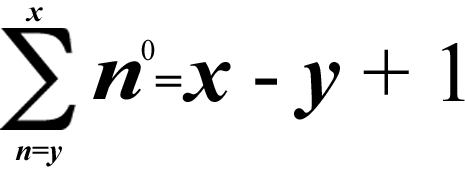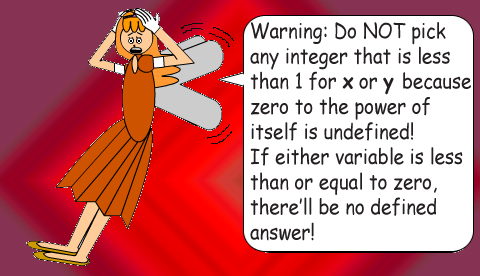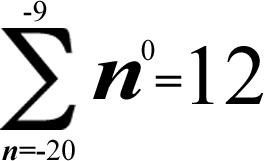# Super Summation 5## (x ≥ y)## Examples:5^0 + 6^0 + 7^0 + 8^0 + 9^0 + 10^0 + 11^0 + 12^0 = 1 + 1 + 1 + 1 + 1 + 1 + 1 + 1 = 12 - 5 + 1 = 8

(Notice that there are 8 1's!)

The caret (^) is the symbol of exponentiation. Raising a non-zero number to the power of zero(0) always gives you 1.

### Note: If x = y, then the summation will add up to 1!### (Actually, if both x & y are negative, the summation will still have a defined result, but it'll always be positive!)(-20)^0 + (-19)^0 + ... + (-9)^0 = 1 + 1 + 1 + 1 + 1 + 1 + 1 + 1 + 1 + 1 + 1 + 1= |(-20) - (-9)| + 1 = 12

(Notice that there are 12 1's!)

Parentheses were put around the negative numbers to prevent some confusion. (-20) - (-9) = -11, but |(-20) - (-9)| = +11; adding +1 to -11 gives you -10, but adding +1 to +11 gives you +12. The vertical line symbols represent absolute value, which is always positive. The function of absolute value makes subtraction commutative! Subtraction without absolute value is not commutative.